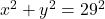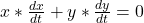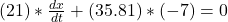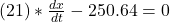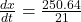## A 29-meter ladder is sliding down a vertical wall so the distance between the top of the ladder and the ground is decreasing at 7 meters per

Question

A 29-meter ladder is sliding down a vertical wall so the distance between the top of the ladder and the ground is decreasing at 7 meters per minute. At a certain instant, the bottom of the ladder is 21 meters from the wall.

in progress 0
5 months 2021-08-23T10:08:11+00:00 1 Answers 61 views 0

## Answers ( )

1. Complete question

A 29-meter ladder is sliding down a vertical wall so the distance between the top of the ladder and the ground is decreasing at 7 meters per minute. At a certain instant, the bottom of the ladder is 21 meters from the wall.

What is the rate of change of the distance between the bottom of the ladder and the wall at that instant(in meters per minute)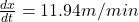Step-by-step explanation:

From the question we are told that

Slant height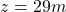Change in Vertical height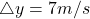Horizontal length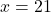Generally in finding the distance form the  top to the bottom of the wall it is mathematically given by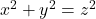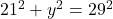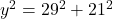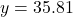Generally solving for the differential equation is mathematically represented as